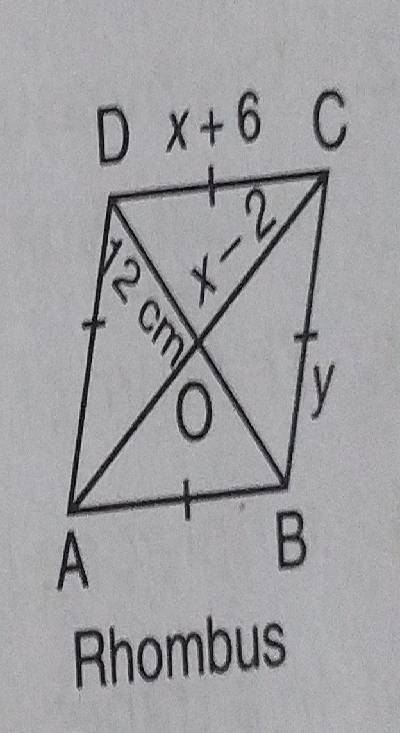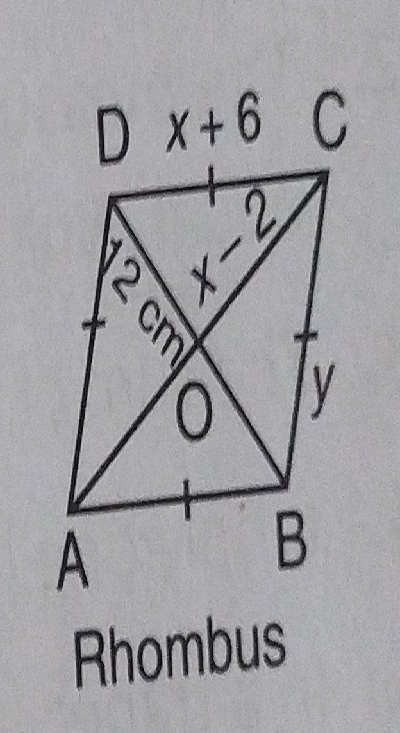"
">

# Find the value of $x$ in rhombus $ABCD$ as shown in the figure."

Given: In the given figure, $ABCD$ is a rhombus and its diagonal intersects at the point $O$.

To do: To find $x$.

Solution:In triangle $\vartriangle OCD$,

$OD=12\ cm,\ OC=( x-2)$ and $CD=( x+6)$

$\angle COD=90^o$    [In a rhombus, diagonals form an angle of $90^o$ at the point of intersection$] On using Pythagoras theorem,$CD^2=OC^2+OD^2\Rightarrow ( x+6)^2=( x-2)^2+12^2\Rightarrow x^2+12x+36=x^2-4x+4+144\Rightarrow 12x+4x=148-36\Rightarrow 16x=112\Rightarrow x=\frac{112}{16}\Rightarrow x=7$Thus,$x=7\$.

Updated on: 10-Oct-2022

26 Views